Get inspired by the success stories of our students in IIT JAM MS, ISI  MStat, CMI MSc DS.  Learn More

# Correlation of two ab(Normals) | ISI MStat 2016 PSB Problem 6This problem is an interesting application of the moment generating function of normal random variable to see how the correlation behaves under monotone function. This is the problem 6 from ISI MStat 2016 PSB.

## Problem

Suppose that random variables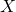and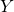jointly have a bivariate normal distribution withand
correlation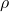. Compute the correlation between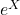and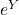.

### Prerequisites

• Correlation Coefficient
• Moment Generating Function
• Moment Generating Function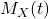of Normal~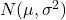is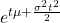.

## Solutionis called the moment generating function.

Now, let's try to calculate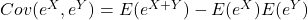For, that we need to have the following in our arsenal.

•~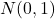•~•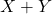~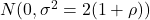[ We will calculate thisjust below ].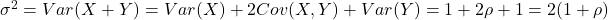.

Now observe the following:

••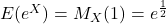••#### Important Observation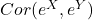and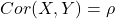always have the same sign. Can you guess why? There is, in fact, a general result, which we will mention soon.

Now, we are left to calculate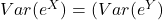.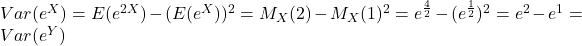.

Therefore,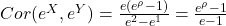.

Observe that the mininum correlation ofandis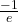.

## Back to the important observationandalways have the same sign. Why is this true?

Because,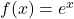is an increasing function. So, ifandare positively correlated then, asincreases,also increases in general, hence,also increases along withhence, the result, which is quite intuitive.

Observe that in place ofif we would have taken, any increasing function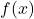, this will be the case. Can you prove it?

Research Problem of the day ( Is the following true? )

Letbe an increasing function of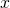, then

•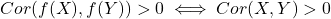•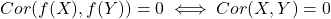•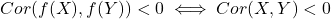This problem is an interesting application of the moment generating function of normal random variable to see how the correlation behaves under monotone function. This is the problem 6 from ISI MStat 2016 PSB.

## Problem

Suppose that random variablesandjointly have a bivariate normal distribution withand
correlation. Compute the correlation betweenand.

### Prerequisites

• Correlation Coefficient
• Moment Generating Function
• Moment Generating Functionof Normal~is.

## Solutionis called the moment generating function.

Now, let's try to calculateFor, that we need to have the following in our arsenal.

•~•~•~[ We will calculate thisjust below ]..

Now observe the following:

••••#### Important Observationandalways have the same sign. Can you guess why? There is, in fact, a general result, which we will mention soon.

Now, we are left to calculate..

Therefore,.

Observe that the mininum correlation ofandis.

## Back to the important observationandalways have the same sign. Why is this true?

Because,is an increasing function. So, ifandare positively correlated then, asincreases,also increases in general, hence,also increases along withhence, the result, which is quite intuitive.

Observe that in place ofif we would have taken, any increasing function, this will be the case. Can you prove it?

Research Problem of the day ( Is the following true? )

Letbe an increasing function of, then

•••This site uses Akismet to reduce spam. Learn how your comment data is processed.

### Knowledge Partner